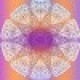Notifications
Clear all

# basics(@doubop)
Core Collaborators

trigonometry: it's about the measurement of the trigones or triangles…cause if you have Three side you have three angle, if you close the form of course.

there's an elementary step that i want to share with you on this chapter. Rules are well known and this often start with the famous pythagorean theorem that we all have suffer to learn his formal definition.

Let's start from the point. with a point alone no relation. You can imagine it  on a line on a plane or a volume space.

let's add a second point.  and try to find a relation between them. we naturally trace a line. because two points can be colinear. in any direction of a 3 d space.

let's add a third point. there is a plane somewhere in where those 3 points are coplanaires. But we want a relation with the first two points. an equal relation because equality is equilibre. Let 's call it c. we want a C as  ac=bc.   it's  a middle point.

but a point can be on many lines. is there just one point that satisfy ac=bc. No the point on the axe of symetria between are answering to this equality. so

As c is center of symetria of  A B . if we want a point D as ac =bc=cd we gonna build a circle center on c. All those points can be a D for a triangle containing BC. it's how when you put 3 you have the circle, and the triangle appears. as circle is an angle of 360, or 2pi rad in math classic, it's opens to all those law of  circles and triangles that can be appears  non intuitive. It's a way to use side and angle, to make a correspondance between straight lines and curve. Or don't forget, that a line can't be straight and curve. it's one or another.

I hope this will be useful for those who are afraid of that's long words and long definition. this first step of reflection, is useful and can be generalize to the sphere. because the center of the circle can be the center of many lines that's satisfy those condition of symetria, and leads to topology. and even to complex number, and to wave modelisation. the rotation of the D point gives all the triangle possible (except for the lenght, which is in fact the homothetie ( 3,4, 5 triangle for example)

of course we can increase the numbers of "gone" or side, and obtain other polygones. But as it works for 3 it works for a circle and all is division. I find it really pretty because as, three is there all this can be possible.

Topic starter Posted : 30/05/2020 10:38 am
Azra Wind liked(@holycowherewego117)
Admin

the first step of reflection ♥

Posted : 01/06/2020 1:09 pm